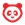cancel
Showing results for
Did you mean:

# How do I create a Numerical Answer quiz question?

## How do I create a Numerical Answer quiz question?

You can create a quiz question that requires a numerical answer, such as questions for a math course.

Note: If you need help adding a mathematical formula in the Rich Content Editor, please see the Math Editor lesson.With the Questions tab  selected inside a new quiz, click the New Question button .

## Set Name, Type, and PointsQuiz questions are not automatically numbered for instructors. To add a custom name to your quiz question, enter the name in the question text field . Custom names can help you identify quiz questions more easily. Regardless of the question name, students always see quiz questions in numerical order (i.e. Question 1, Question 2).

Click the drop-down menu and select the Numerical Answer question type .

Enter number of points the question is worth (quiz totals are calculated based on combined total of questions) .

Note: Quiz point values support up to two decimal places. Entering more than two decimal places will round the point value to the nearest hundredth.

## Enter Question DetailsType your question into the Rich Content Editor question box. You can also use the Rich Content Editor to include video, images, math equations, or flash activities.Exact answer : Enter the exact answer with the exact error margin allowed. For example, if the answer were 2.12 with a 0.01 margin of error, the quiz accepts any response between 2.11 and 2.13 (inclusively). By default, the margin is 0.

Answer in the Range : Enter the range of answers that can be accepted as correct, such as answers between 8 and 10.

Answer with Precision : Enter the answer with the precision number. The precision number is how many digits are expected in the answer. Precision answers can be negative numbers and may include trailing zeroes. However, student responses will be marked as correct if they omit the trailing zeroes, as long as all preceding digits are correct.

By default, the precision number is 10, but answers can be rounded to a precision of up to 16 in standard or exponential format (e.g. 1e+26). Answers are accepted with or without the e exponent and are formatted according to the configured precision. Any numerical entries past 16 are truncated for grading and comparison. If any of the potential answers to a numerical question are a precision answer, then the student answer fields will accept precision answers.

If none of the possible answers are precision answers, the student answer fields round to four decimal places.

## Enter Feedback TextYou can choose to give students feedback in the answer comments fields. To leave feedback for a specific answer, click the comment field beneath the answer .

You can also create general answer comments for the quiz question as a whole . Students who select the correct answer will see the feedback in the green comment field. Students who select any incorrect answer will see the feedback in the red comment field. All students will see the feedback in the blue comment field.

Note: Any applicable feedback is visible as soon as a quiz is submitted and cannot be hidden from students.Each text box can be expanded in size by clicking and dragging the handle at the bottom-right of the text box.

## Update QuestionClick the Update Question button.

## Student View for Numerical Answer QuestionThis is what students see when they encounter a Numerical Answer question in a quiz.If you allow students to see the correct answers, they will see correct responses in gray . If the answer was correct, the student will see a green flag  indicating the answer was correct.If you allow students to see the correct answers, they will see correct responses and allowable margin error . If the answer was incorrect, the student will see a red flag indicating the answer was incorrect .

#### These comments are posted to the global Canvas Community,